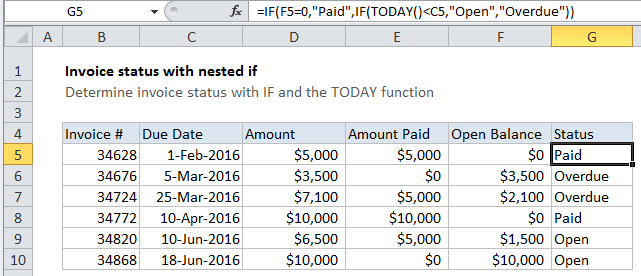## Excel Office

Excel How Tos, Tutorials, Tips & Tricks, Shortcuts

# Invoice status with nested if in Excel

This tutorial shows how to calculate Invoice status with nested if in Excel using the example below;

## Formula

`=IF(balance=0,"Paid",IF(current_date<due_date,"Open","Overdue"))`## Explanation

To determine invoice status (i.e. paid, open, overdue), you can use a nested IF formula and the TODAY function.

In the example shown, the formula in G5 is:

`=IF(F5=0,"Paid",IF(TODAY()<C5,"Open","Overdue"))`

### How this formula works

Note: the “current date” (i.e. today) for this example is May 31, 2016

Also See:   How to strip numeric characters from cell in Excel

With nested IF statements, the flow is from outer IF statements to inner IF statements, and the challenge is always to construct the flow so that the formula returns a logically correct result.

Here, the outermost IF tests first to see if the balance is zero:

`=IF(F5=0`

If TRUE, the formula returns “Paid”.

Also See:   Excel Data validation with conditional list

If not, the result of the first IF is FALSE, and another IF statement is run. This one checks to see if TODAY() is less than the due date in column C:

`IF(TODAY()<C5`

If TRUE, the formula returns “Open”.

If FALSE, the formula returns “Overdue”.